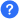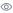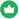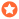In Swift programming language, there are many inbuilt functions that are used to perform various mathematical operations.

This study list contains solved Swift mathematical functions programs, practice these programs to learn the concept of mathematical operations using the mathematical library functions, these programs contain the solved code, outputs, and the detailed explanation of the statements, functions used in the Swift mathematical functions programs.

### questions

Title
###### total questions: 23Questions

##### 23Views

##### 161